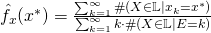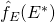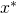# 2. Coding the “Educated Guess Procedure”

## 1. Perform the Analyze

To start with, we load the “rockyou.txt.tar.gz” password list using wget. I’m not sure if it is legal to provide a link for the list, therefore just ask a search engine 😉 . The next step is to extract the file sudo tar -zxvf rockyou.txt.tar.gz and copy the data into the Hadoop File system. We create a new folder hadoop fs -mkdir /passwords and copy the data hadoop fs -copyFromLocal rockyou.txt /passwords to this new folder. To test the script I also loaded the rockyou-10.txt file which contains a sample. We will now compute an estimator for the density of the word length given byand accordinglyas an estimator for the density for a given letter (see this post for notation details). The density are then saved to a CSV file and the 10 elements with the highest probability are printed out.

from pyspark import SparkContext
#sc =SparkContext()

###To check our code we first load a subsample
###Explore Passwort length
#Compute the length of the Passwords
leng= passwords.map(lambda x : len(x) )
##Compute Props
#Count the amount of each length
#divide by the total amount of the word length to get a density
length_dens=absv.map(lambda (y,x): (y,true_divide(x,pws_cnt))).cache()
#save the densitys to a text file
length_dens.saveAsTextFile("length_dens.csv")
#print the 10 most popular word length
print length_dens.takeOrdered(10, lambda (k, v): -v)

##Compute Props for letter
#construct one long array of letters
flatpw=passwords.map(lambda line : list(line) ).flatMap(lambda x:x)
#count the length of this array
word_cnt= flatpw.count()
#count the amount each letter
#divide by the total letters to get a density
word_dens=abswr.map(lambda (y,x): (y,true_divide(x,word_cnt))).cache()
#save the densitys to a text file
word_dens.saveAsTextFile("word_dens.csv")
#print the 10 most popular letters
print word_dens.takeOrdered(10, lambda (k, v): -v)


What we learn from this toy experiment?8 0.20684851660833842 7 0.17479055053644313 9 0.15278606111615334 10 0.14040993444754818 6 0.13589095556583755 11 0.060365058370201986 12 0.038697146501374652 13 0.025382464825449893 5 0.018100454735234143 14 0.017306904141137815

For the letter density we derivea 0.070450032335993312 e 0.057498677721715831 1 0.05366393124621479 0 0.04574238186102738 i 0.044320375895981298 2 0.04173564796751799 o 0.041303826710886733 n 0.038522687445766347 r 0.036524316843870627 l 0.035604072994829351

So if you have no clue about some password, try a password of length 8 with a lot of a’s and e’s in it 😉

### 1 thought on “2. Coding the “Educated Guess Procedure””

This site uses Akismet to reduce spam. Learn how your comment data is processed.